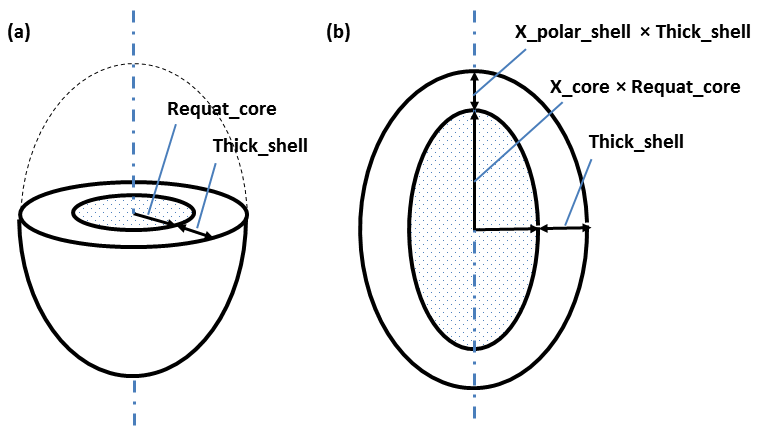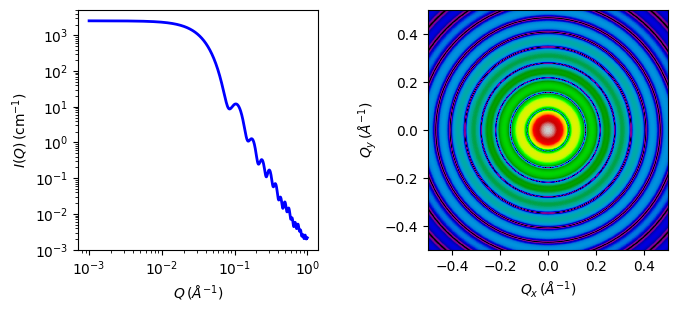# core_shell_ellipsoid

Form factor for an spheroid ellipsoid particle with a core shell structure.

Parameter Description Units Default value
scale Scale factor or Volume fraction None 1
background Source background cm-1 0.001
x_core axial ratio of core, X = r_polar/r_equatorial None 3
thick_shell thickness of shell at equator 30
x_polar_shell ratio of thickness of shell at pole to that at equator None 1
sld_core Core scattering length density 10-6-2 2
sld_shell Shell scattering length density 10-6-2 1
sld_solvent Solvent scattering length density 10-6-2 6.3
theta elipsoid axis to beam angle degree 0
phi rotation about beam degree 0

The returned value is scaled to units of cm-1 sr-1, absolute scale.

Definition

Parameters for this model are the core axial ratio $$X_{core}$$ and a shell thickness $$t_{shell}$$, which are more often what we would like to determine and make the model better behaved, particularly when polydispersity is applied, than the four independent radii used in the original parameterization of this model.The geometric parameters of this model are shown in the diagram above, which shows (a) a cut through at the circular equator and (b) a cross section through the poles, of a prolate ellipsoid.

When $$X_{core}$$ < 1 the core is oblate; when $$X_{core}$$ > 1 it is prolate. $$X_{core}$$ = 1 is a spherical core.

For a fixed shell thickness $$X_{polar shell}$$ = 1, to scale $$t_{shell}$$ pro-rata with the radius set or constrain $$X_{polar shell}$$ = $$X_{core}$$.

Note

When including an $$S(q)$$, the radius in $$S(q)$$ is calculated to be that of a sphere with the same 2nd virial coefficient of the outer surface of the ellipsoid. This may have some undesirable effects if the aspect ratio of the ellipsoid is large (ie, if $$X << 1$$ or $$X >> 1$$), when the $$S(q)$$ - which assumes spheres - will not in any case be valid. Generating a custom product model will enable separate effective volume fraction and effective radius in the $$S(q)$$.

If SAS data are in absolute units, and the SLDs are correct, then scale should be the total volume fraction of the “outer particle”. When $$S(q)$$ is introduced this moves to the $$S(q)$$ volume fraction, and scale should then be 1.0, or contain some other units conversion factor (for example, if you have SAXS data).

The calculation of intensity follows that for the solid ellipsoid, but with separate terms for the core-shell and shell-solvent boundaries.

$P(q,\alpha) = \frac{\text{scale}}{V} F^2(q,\alpha) + \text{background}$

where

\begin{align*} F(q,\alpha) = &f(q,R_e,R_e.x_{core},\alpha) \\ &+ f(q,R_e + t_{shell}, R_e.x_{core} + t_{shell}.x_{polar shell},\alpha) \end{align*}

where

$f(q,R_e,R_p,\alpha) = \frac{3 \Delta \rho V (\sin[qr(R_p,R_e,\alpha)] - \cos[qr(R_p,R_e,\alpha)])} {[qr(R_p,R_e,\alpha)]^3}$

and

$r(R_e,R_p,\alpha) = \left[ R_e^2 \sin^2 \alpha + R_p^2 \cos^2 \alpha \right]^{1/2}$

$$\alpha$$ is the angle between the axis of the ellipsoid and $$\vec q$$, $$V = (4/3)\pi R_pR_e^2$$ is the volume of the ellipsoid , $$R_p$$ is the polar radius along the rotational axis of the ellipsoid, $$R_e$$ is the equatorial radius perpendicular to the rotational axis of the ellipsoid, $$t_{shell}$$ is the thickness of the shell at the equator, and $$\Delta \rho$$ (the contrast) is the scattering length density difference, either $$(\rho_{core} - \rho_{shell})$$ or $$(\rho_{shell} - \rho_{solvent})$$.

For randomly oriented particles:

$F^2(q)=\int_{0}^{\pi/2}{F^2(q,\alpha)\sin(\alpha)d\alpha}$

For oriented ellipsoids the theta, phi and psi orientation parameters will appear when fitting 2D data, see the elliptical_cylinder model for further information.Fig. 39 1D and 2D plots corresponding to the default parameters of the model.

Source

core_shell_ellipsoid.py $$\ \star\$$ core_shell_ellipsoid.c $$\ \star\$$ gauss76.c $$\ \star\$$ sas_3j1x_x.c

References see for example:

1. Kotlarchyk, M.; Chen, S.-H. J. Chem. Phys., 1983, 79, 2461
2. Berr, S. J. Phys. Chem., 1987, 91, 4760

Authorship and Verification

• Author: NIST IGOR/DANSE Date: pre 2010